# TDS on Salary Calculation | Tax Deduction on SalaryOne of the important questions on my YouTube Channel is What is TDS on Salary and How to Calculate TDS on Salary?

TDS full form is Tax Deduction at Source. Every Salary employee is liable to pay Income Tax based on the Income Tax Calculation. But Income Tax need not be voluntarily paid by individuals.

It can also be deducted from Salary before receiving the Salary credit message in your Bank account. This is called TDS or Tax Deduction at Source.

TDS on Salary is deducted every month, which will be equivalent to your total income tax you are liable to pay in entire financial year.

Below is the video on How TDS is calculated on Salary with examples.

## TDS on Salary Calculator Video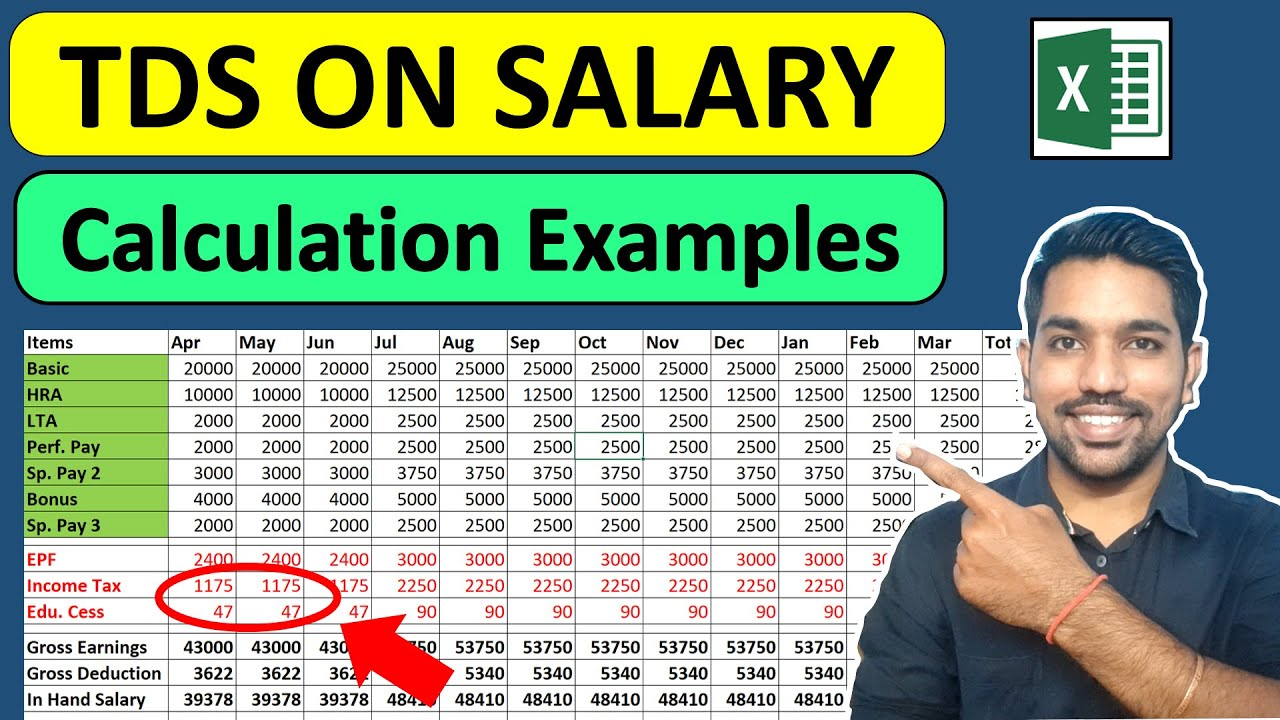Watch more Excel Videos Here

As seen in above video, it is very important to understand that TDS on Salary is deducted with every month salary.

Your total income tax in financial year is divided by remaining months in the year to get every month TDS deduction from Salary.

Let us understand all this is more details.

## What is TDS and Why it is Deducted?

TDS or Tax Deduction at Source from Salary is a way to pay your income tax via your Salary credits. Your organization (or company) is liable to pay income tax on your behalf if you fall in Income Tax Bracket.

As a responsible citizen of India, you have to make sure that correct income tax is deducted from your salary and that is the reason why we should file our Income Tax Returns every year, that ensures correct tax is paid by us.

Due to above reasons, TDS is calculated and deducted every month from our salary to pay the income tax we are liable to pay.

The main advantage of paying income tax in this way as an individual is that we pay a small fraction of tax every month, compared to paying huge amount of tax at one go in the end of financial year.

Let us now understand the components of Payslip first before we move to the example of TDS on Salary Calculation.

## Salary Slip components

Below is the screenshot of a salary payslip for reference:

As we can see above, Earnings are displayed on left hand side (LHS) and Deductions are displayed on right hand side (RHS).

The total of LHS will give us the in-hand salary, whereas the total on RHS will give us total deductions. Sum of both these totals will give us Gross Income.

It is very important to calculate Taxable Income in order to calculate income tax that will help us know TDS deduction every month.

We will see how to calculated Taxable Income now. First we will calculate Gross Earnings for a month.

### Calculating Gross Earnings

So using numbers mentioned on LHS of above Salary payslip, we get the total Gross Earnings or in-hand salary or the amount that will be credited to our bank account is Rs. 54,920

This is the Gross Earnings for a month. Let’s calculate Gross Deductions for a month.

### Calculating Gross Deductions

By using numbers on RHS, we get the total deductions for a month as Rs. 4,050.

This is the Gross Deductions for a month.

### Calculating Gross Income

Addition of both earnings and deductions will be Gross Income for the month, which will be Rs. 54,920 + Rs. 4,050 = Rs. 58,970.

Using this monthly Gross Income, we get yearly Gross Income as Rs. 58,970 * 12 = Rs. 7,07,640

But it is not necessary that we have to pay income tax on this entire amount.

We can have deductions including Provident Fund (as shown in salary slip above), public provident fund, life insurance premiums, 5 year Fixed Deposits, and many other Tax Saving Options to Save Income Tax.

Let us now calculate Taxable Income.

### Calculating Taxable Income

The basic formula to calculate Taxable Income is to remove al deductions or investments made from gross income. This will give us taxable income on which income tax will be calculated.

Taxable Income = Gross Income – Deductions

Let us calculate Taxable Income on our example above where:

• Yearly Gross Income = Rs. 7,07,640
• Yearly Gross Deductions = Rs. 29,520 (Rs. 2460 of Provident Fund * 12 months)

Notice that we have only considered Provident Fund as the deduction above. Other deductions cannot be claimed to reduce taxable income.

We can only use mentioned deduction options to reduce taxable income to save income tax and hence, not all deductions are considered as non taxable.

So considering above numbers, we also have to consider Standard Deduction of Rs. 50,000 if you are a salaried employee (or pensioner), we get below taxable income based on Old and New Tax Regime:

• Taxable Income (Old Tax Regime) = Rs. 6,28,120 (Rs. 7,07,640 – Rs. 29,520 – Rs. 50,000)
• Taxable Income (New Tax Regime) = Rs. 7,07,640

For New Tax Regime, there are no deductions that we can claim to reduce taxable income. Hence, gross income will be our taxable income in new tax regime.

Since now we have Taxable Income (and gross income as well), we can easily calculate Income Tax using online calculator here.

IMPORTANT: You can also use other investment options to reduce your Taxable income to save your income tax under Old Tax Regime. Watch below video to know more tax saving options.

### All Deductions in Section 80 – Video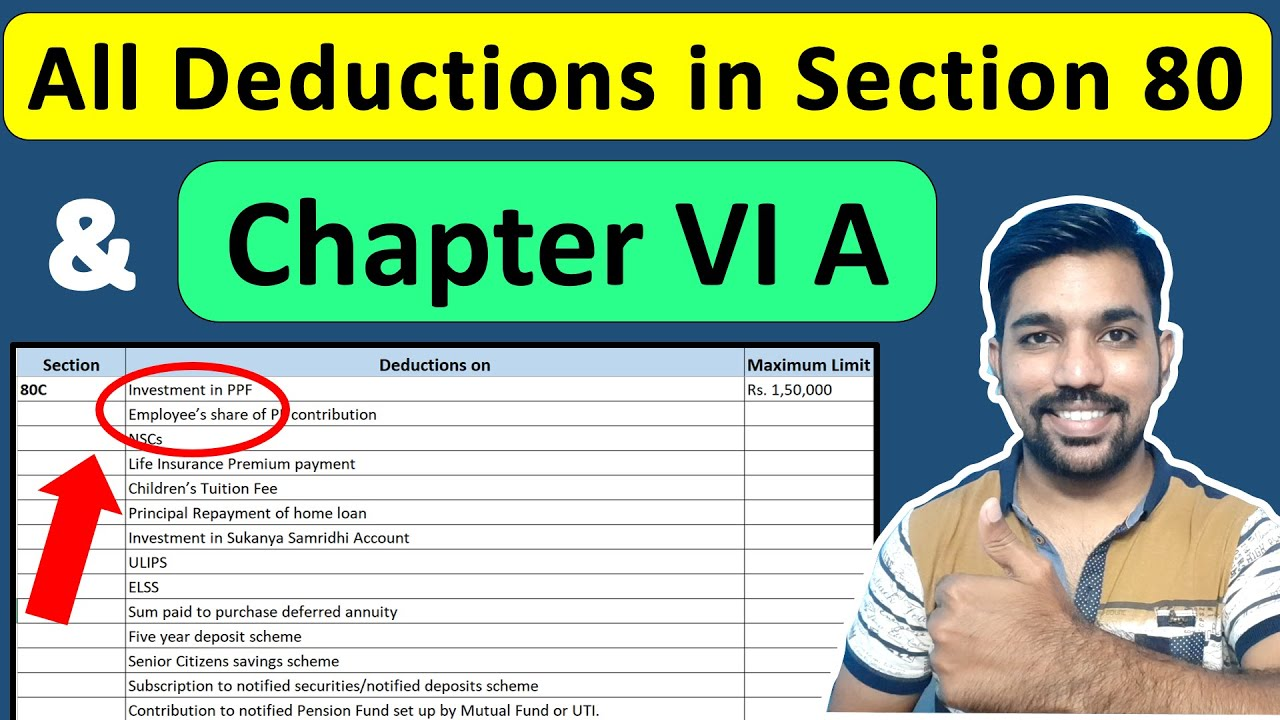## Income Tax Calculation on Gross Earnings

Let us now calculate Income Tax using our Income Tax Calculator.

We will provide Gross Income in Income Section and appropriate Deductions in Deductions Section. Standard Deduction of Rs. 50,000 will be applied automatically based on selection of option – Salaried or Pensioner.

As seen above, we get the income tax for mentioned gross income and deductions as:

• Old Tax Regime = Rs. 39,649
• New Tax Regime = Rs. 34,595

Since we have the option to choose between Old Tax Regime and New Tax Regime, it is clear that we should select new Tax Regime to pay income tax as we will be paying less income tax with new tax regime in this example.

But, if you have more deduction options to be used, you should definitely use under Deduction Section to save more income tax with Old Tax Regime and it can definitely be reduced.

One of the tax saving options is Home Loan Tax Benefits you get if you have Home Loan, so you can check out below Home Loan Tax Benefits Video as well.

### Home Loan Tax Benefits – Video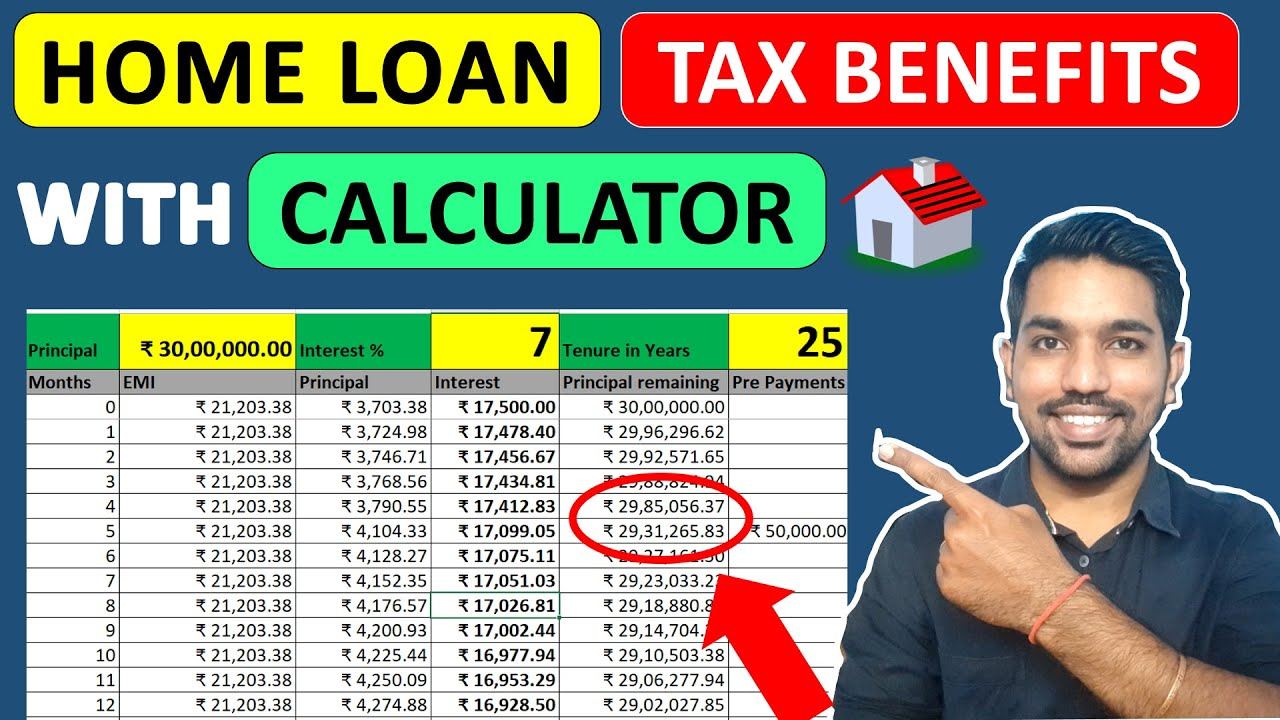Watch more Excel Videos Here

## Calculation of TDS on Salary Example

Now the very important step is to calculate TDS on Salary using this income tax that we have calculated.

Since in above calculation, we have realized that new tax regime is the once that we should go with, since it will help us pay less income tax considering the deduction options we have used, we will take income tax of Rs. 34,595 of new tax regime as example to calculate every month TDS.

As mentioned above, this total income tax of entire financial year need to be paid by you every month in the form of TDS from your salary. Let us check this with our example:

### Creating Excel with Payslip components

In our example we will use an excel document to calculate every month TDS on Salary, which will mention different components of salary payslip as well. Below is the screenshot of the excel:

As seen above, in green background we have the salary components belonging to earnings (in hand Salary), in red we have the deductions. We only have to consider Provident fund deduction to calculate taxable income due to above mentioned reasons.

### Calculating monthly Income Tax and Education Cess

Now to calculate income tax and Education cess every month from salary, we already have the total income tax to be paid (Rs. 34,595) based on new tax regime we selected.

Now this income tax is the combination of Income Tax and Education cess. We need to separate both these components.

So we can use our Income Tax Excel Calculator, and below is the calculations:

As highlighted in yellow above, the income tax is Rs. 33,264 and Education Cess is Rs. 1330.56, which makes total income tax as Rs. 34,595 based on new tax regime. So now we have separate components.

Based on above numbers, we will have every month TDS calculation with 2 components as:

• Every month Income Tax = Rs. 33,264 / 12 months = Rs. 2,772
• Every month Education Cess = Rs. 1330.56 / 12 months = Rs. 111
• Every month TDS deduction = Rs. 2,772 + Rs. 111 = Rs. 2,883

So Rs. 2,883 is the approximate every month TDS to be deducted from your salary. This when multiplied by 12 months will give us a total income tax of approximately Rs. 34,596.

### Calculating in-hand Salary after TDS Deduction

Ans this is the way TDS is calculated and deducted every month. Your in-hand salary will be calculated accordingly by reducing this every month TDS as shown in the screenshot below:

As seen above, in-hand salary is reduced from Rs. 54,920 to Rs. 52,037 due to the TDS of Rs. 2,883 every month that is deducted from salary.

This is how TDS will be calculated and deducted every month.

Please note that these are approximate figures and we have considered same salary throughout the financial year. In real scenario you might switch your jobs or get a raise which will impact your total income tax and TDS deduction accordingly.

So you have to consider those factors as well. Let us consider another example of TDS calculation with salary increment.

## TDS on Salary Increment

Let’s consider 10% increment in all salary components of above example from the month of July to March. Below is the screenshot with updated salary components and deductions:

Below are some points to consider in this salary increment:

• 10% increase in every payslip component from July to March
• EPF contribution is also increased from July
• As a result, gross income is increased to Rs. 7,59,282
• Total PF Contribution for deduction = Rs. 31,734
• In hand salary is also updated as shown above

Let us now calculate income tax based on gross income = Rs. 7,59,282 and deductions = Rs. 31,734 using income tax calculator:

So we get the total income tax as:

• Income Tax (Old Tax Regime) = Rs. 49,930
• Income Tax (New Tax Regime) = Rs. 40,448

It would be wise to choose new tax regime here to pay our income tax since it will be less compared to old tax regime.

Let’s verify these numbers in our excel calculator as well. We consider Provident Fund and Standard Deduction of Rs. 50,000 in total investments:

As seen above, we get income tax and education cess components. But out of this, we already paid total income tax of Rs. 8,649 (Rs. 8316 as income tax and Rs. 333 as education cess)between months April to June.

So we have to subtract these numbers from updated income tax numbers:

• Every month Income Tax = (Rs. 38,892 – Rs. 8316) / 9 months = Rs. 3,397
• Every month Education Cess = (Rs. 1556.692 – Rs. 333) / 9 months = Rs. 136
• Every month TDS deduction = Rs. 3,397 + Rs. 136 = Rs. 3,533 (between July to March)

So Rs. 3,533 is the approximate every month TDS to be deducted from your salary between July to March. This when added to April to June Income Tax will give us a total income tax of approximately Rs. 40,448 that we calculated as total income tax on online calculator and excel calculator.

Let us put these numbers in TDS excel and get the final TDS deductions for all months after salary increment:

## Conclusion

So this is the way we calculate TDS deduction on Salary every month. Please note that these are approximate figures we calculated, the numbers might slightly differ in real scenario when there are multiple salary increments and bonuses to be considered.

Below is the Summary to calculate TDS on Salary:

• Calculate your projected gross income for the financial year
• Calculate projected Deductions that are non taxable
• Get Taxable Income for the financial year
• Calculate Income Tax using online calculator or excel calculator
• Divide income tax and education cess by remaining number of months
• Above step will give you TDS deduction for every month in financial year

Comment below if you have any queries or watch this video to see all steps on TDS Calculation on Salary. Let us check some frequently asked questions now.

### How much is TDS for Salary?

Based on the Gross Income and deductions that can be claimed, we have to calculate income tax using the income tax calculator mentioned above.

This income tax will then be divided by remaining months of financial year to get TDS deduction on Salary.

### What is TDS rate for 50000 Salary?

Total Income Tax (or TDS) on Salary of Rs. 50,000 will be approximately Rs. 23,400 based on Old and New Tax Regime. This TDS or income tax can be reduced by using various Tax Saving Options.

You can use the above mentioned income tax calculator to check income tax on your gross income and deductions.

### Is TDS applicable on 30000 Salary?

With Rs. 30,000 as Salary, total income in financial year will be approximately Rs. 3,60,000. And as we have already seen in this YouTube Video, we need not have to pay any Tax on income below Rs. 5 Lacs in a Financial year.

So no TDS or income tax is applicable on 30000 Salary, provided there are no other sources of income and total taxable income in financial year is below Rs. 5 Lacs.

### Who Should pay TDS on Salary?

Anyone who earns considerable amount of income every financial year, such that the taxable income goes above Rs. 5 Lacs in a year, is liable to pay TDS on Salary or income tax.

Also you can watch this video on income tax calculation: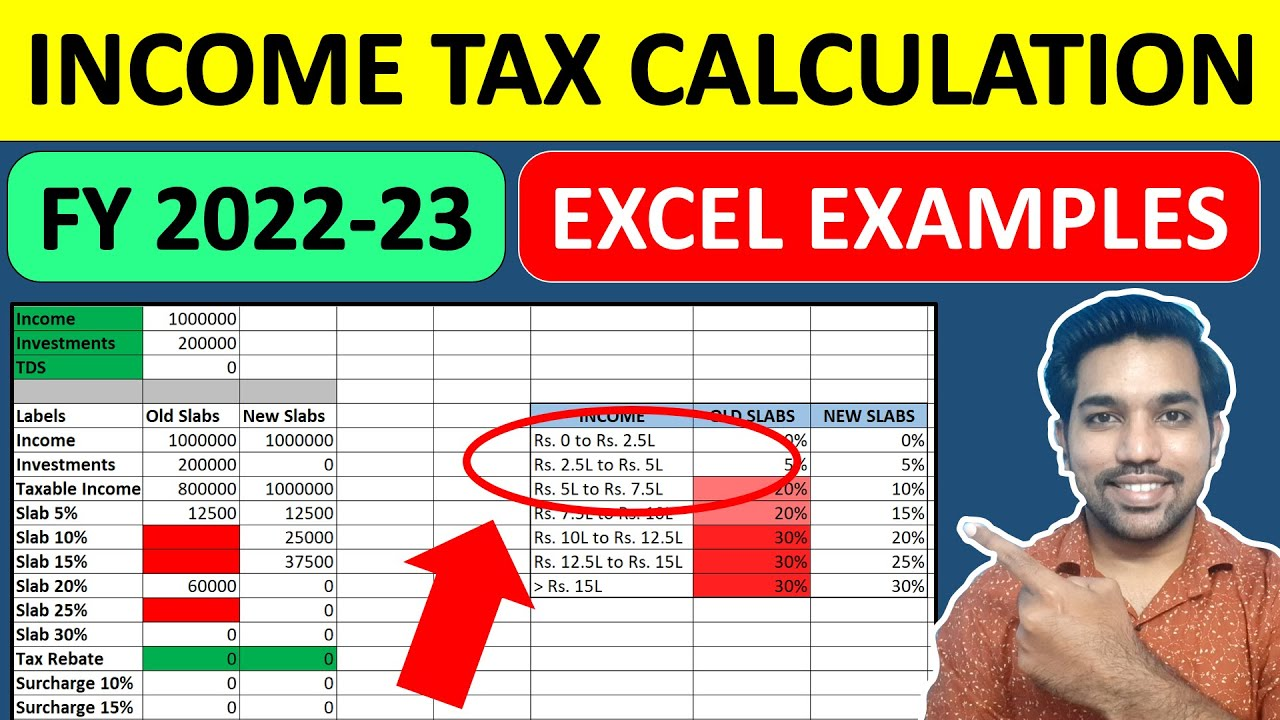### How we can save TDS on Salary?

You can easily save TDS on Salary via Old Tax Regime by using various Tax Saving options mentioned here.

Please note that these deduction options are not allowed in new tax regime and cannot be claimed to save income tax if you opt for New tax regime.

### How is Tax Calculated on Salary?

Based on this income tax calculation article, below are the summarized steps to calculate your income tax:

• Choose between Old and New Tax Regime
• Calculate Income Tax based on Tax Regime using income tax calculator

Watch this video to know all steps in action: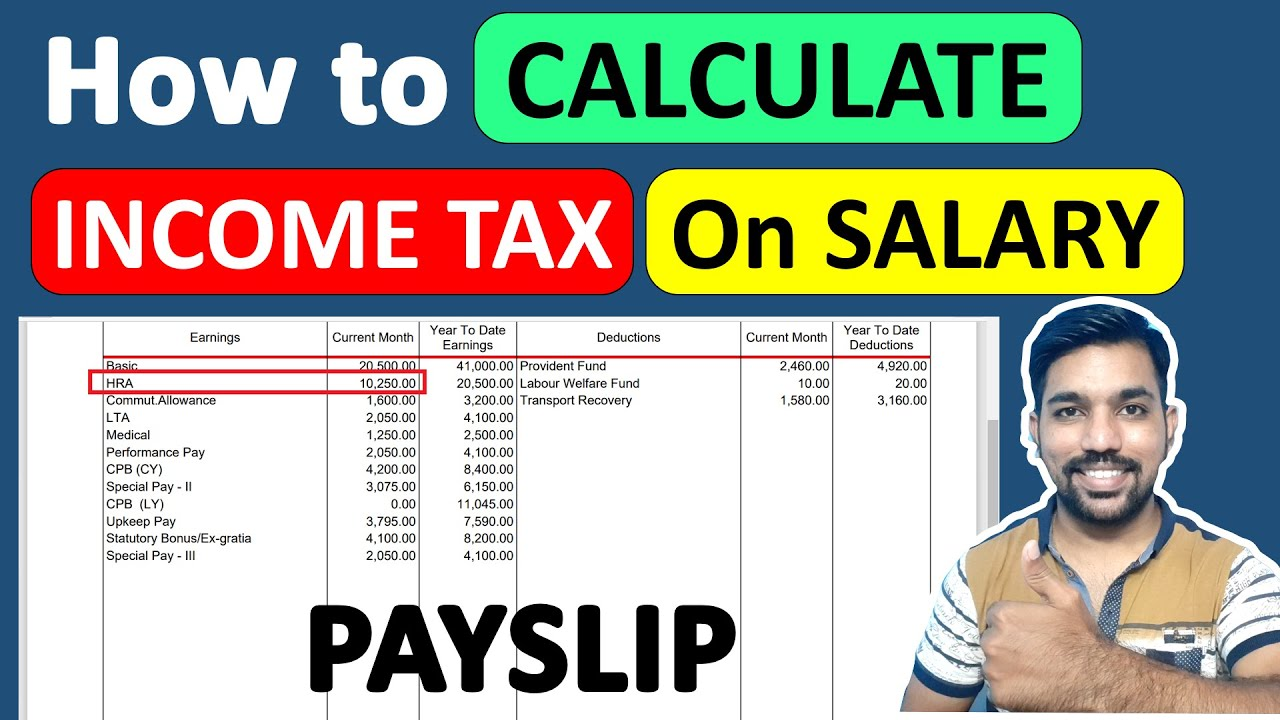Found this Helpful? DONATE any amount to see more useful Content. Scan below QR code using any UPI App!

UPI ID: abhilashgupta8149-1@okhdfcbank

Verify that you are “Paying Abhilash Gupta” before making the transaction so that it reaches me. It makes my Day 🙂

Thank you for Donating. Stay Tuned!

## Income Tax Calculator App – FinCalC

For Income Tax Calculation on your mobile device, you can Download my Android App “FinCalC” which I have developed for you to make your income tax calculation easy.

### What you can do with this mobile App?

• Calculate Income Tax for new FY 2023-24 and previous FY 2022-23
• Enter estimated Investments to check income tax with Old and New Tax Regime
• Save income tax details and track regularly
• Know how much to invest more to save income tax
• More calculators including PPF, SIP returns, Savings account interest and lot more